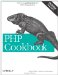# Recipe 2.1. Checking Whether a Variable Contains a Valid Number

#### 2.1.1. Problem

You want to ensure that a variable contains a number, even if it's typed as a string. Alternatively, you want to check if a variable is not only a number, but is also specifically typed as a one.

#### 2.1.2. Solution

Use is_numeric( ) to discover whether a variable contains a number:

`<?php if (is_numeric(5))          { /* true  */ } if (is_numeric('5'))        { /* true  */ } if (is_numeric("05"))       { /* true  */ } if (is_numeric('five'))     { /* false */ } if (is_numeric(0xDECAFBAD)) { /* true  */ } if (is_numeric("10e200"))   { /* true  */ } ?>`

#### 2.1.3. Discussion

Numbers come in all shapes and sizes. You cannot assume that something is a number simply because it only contains the characters 0 through 9. What about decimal points, or negative signs? You can't simply add them into the mix because the negative must come at the front, and you can only have one decimal point. And then there's hexadecimal numbers and scientific notation.

Instead of rolling your own function, use is_numeric( ) to check whether a variable holds something that's either an actual number (as in it's typed as an integer or floating point), or it's a string containing characters that can be translated into a number.

There's an actual difference here. Technically, the integer 5 and the string 5 aren't the same in PHP. However, most of the time you won't actually be concerned about the distinction, which is why the behavior of is_numeric( ) is useful.

Helpfully, is_numeric( ) properly parses decimal numbers, such as 5.1; however, numbers with thousands separators, such as 5,100, cause is_numeric( ) to return false.

To strip the thousands separators from your number before calling is_numeric( ), use str_replace( ):

`<?php is_numeric(str_replace(\$number, ',', '')); ?>`

To check if your number is a specific type, there are a variety of related functions with self-explanatory names: is_float( ) (or is_double( ) or is_real( ); they're all the same) and is_int( ) (or is_integer( ) or is_long( )).

To validate input data, use the techniques from Recipe 9.3 instead of is_numeric( ). That recipe describes how to check for positive or negative integers, decimal numbers, and a handful of other formats.

Recipe 9.3 for validating numeric user input; documentation on is_numeric( ) at http://www.php.net/is-numeric and str_replace( ) at http://www.php.net/str-replace.PHP Cookbook: Solutions and Examples for PHP Programmers
ISBN: 0596101015
EAN: 2147483647
Year: 2006
Pages: 445

Similar book on Amazon